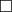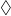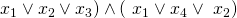# Tag Archives: Difficulty 2

## Modal Logic S5-Satisfiability

One of the things that strikes me about the logic chapter is that the problems all seem to be either “trivial” or “really, really, hard”.  I just hope I’m classifying them correctly.  Here’s one that I think is a trivial one.

The problem: Modal Logic S5-Satisfiability.  This is problem LO13 in the appendix.

The description:  Given a set of variables, and a modal logic formula over those variables, is it “S5-Satisfiable”?  I think that “S5-Satisfiable” is just the regular notion of satisfiability extended to the modal operators.

Example/Reduction: I think all we need to know about Modal Logic for our purposes can be found in from this Wikipedia article. In it, they say that Modal Logic extends propositional or predicate logic by adding two new operations:

•which means “Necessarily”.  SoP means “it is necessary that P is true”.
•which means “Possibly”.  SoP means “it is possible that P is true”.

Since you can definein terms of, G&J just have the diamond symbol in the language.

People use Modal logic to think about “worlds” in which facts are necessarily or possibly true, and different agents know different things.  I talk about it (vaguely) when I teach Artificial Intelligence because it can model things agents know, and things agents know that other agents know, and so on.  (The Russell and Norvig AI book has a fun example about how Superman knows that Clark Kent is Superman, but Lois Lane doesn’t know.  And Superman knows that Lois doesn’t know.  But he knows that Lois knows that someone is Superman).

Notice, though, that for our purposes, we don’t need the modal operators.  So, for example, a regular 3SAT formula: (is a modal logic formula (and, in this case, is true by setting all 4 variables to true).  So the reduction from 3SAT is just “Take a 3Sat formula, keep it the same, call it a modal logic formula.  Obviously, this is satisfiable iff the original (unchanged) formula is”.

Difficulty: 2.  Maybe it should be 1, but you need to at least think a little about what you’re leaving out.